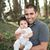# Alok Menghrajani

Previously: security engineer at Square, co-author of HackLang, put the 's' in https at Facebook. Maker of CTFs.

This blog does not use any tracking cookies and does not serve any ads. Enjoy your anonymity; I have no idea who you are, where you came from, and where you are headed to. Let's dream of an Internet from times past.

Can you solve the following popular logic puzzle? How about writting a program to solve it?

Design a 3-input 3-output logic circuit that negates the 3 signals. You have an infinite supply of AND and OR gates but only two NOT gates.

Keep reading to see how to find a solution using Prolog, a logic programming language.

Prolog is a programming environment where you provide rules and then query for results. Search related problems can be solved using just a few lines of code. For example, the following rules say that either `foo(4, 3) is true` or `foo(X, 2) is true when X is 0, 1 or 2`:

```foo(4, 3).
foo(X, Y) :-
member(X, [0, 1, 2]),
Y=2.
```
You can then run queries by providing some, all or no input:
```?- foo(0, 0).
false.

?- foo(1, 2).
true.

?- foo(2, A).
A = 2.

?- foo(A, 2).
A = 0
A = 1
A = 2.

?- foo(A, B).
A = 4, B = 3
A = 0, B = 2
A = 1, B = 2
A = 2, B = 2
```

Usually, the last parameter of a function is the output. The nice thing about Prolog is that you don't need to think in terms of inputs and outputs. You can use functions in either "direction". I.e. given some inputs, you can compute an output, or given an output, you can search for a valid input (as long as the search is bounded).

This power comes with a caveat: Prolog code usually runs slow since the program is branching into various possible paths until a solution is found.

The first step to solve the 3 signals negation puzzle is to define a signal. A simple way is to have each signal be a tuple(int, expr). The integer represents the value of the signal for all 8 possible combinations of values 3 signals can have:

```S0 = [(0b11110000, a), (0b11001100, b), (0b10101010, c)].
```

And we can then define our three operators. Prolog has some weird syntax for bitwise operators:

```make_signal((V1, E1), (V2, E2), or, NewSignal) :-
O is V1 \/ V2,
NewSignal = (O, or(E1, E2)).

make_signal((V1, E1), (V2, E2), and, NewSignal) :-
O is V1 /\ V2,
NewSignal = (O, and(E1, E2)).

make_signal((V, E), not, NewSignal) :-
O is \V /\ 255,
NewSignal = (O, not(E)).
```

Given that we can use as many AND/OR gates as we want, we'll expand `S0` with all the possible AND/OR gates. Doing this helps run the code faster, but does not guarantee that we will find the simplest solution:

```% Given two signals, create a new signal using 'OR' and 'AND' operations.
op(SignalsIn, S1, S2, Op, SignalsOut) :-
% S1, S2 must be in the input set.
member(S1, SignalsIn),
member(S2, SignalsIn),
% Create a new signal.
make_signal(S1, S2, Op, (V, E)),
(member((V, _), SignalsIn) ->
% The resulting signal already exists, bail.
false;
% Add the new signal to the list of outputs.
append(SignalsIn, [(V, E)], SignalsOut)).

% Calls the above function as long as it returns true.
combination_and_or(Sin, Sout) :-
(op(Sin, _, _, _, S) ->
% The list changed, so call combination_and_or recursively.
combination_and_or(S, Sout);
% We are done.
Sout = Sin).
```

Handling the NOT gates is easy, we simply use `_` and let Prolog figure out the right values:

```op(SignalsIn, S, not, SignalsOut) :-
member(S, SignalsIn),
make_signal(S, not, (V, E)),
(member((V, _), SignalsIn) ->
% The resulting signal already exists, bail.
false;
% Add the new signal to the list of outputs.
append(SignalsIn, [(V, E)], SignalsOut)).

solve :-
% Source signals
S0 = [(0b11110000, a), (0b11001100, b), (0b10101010, c)],

% Target signals
T1 = 0b00001111, T2 = 0b00110011, T3 = 0b01010101,

combination_and_or(S0, S1),
op(S1, _, not, S2),
combination_and_or(S2, S3),
op(S3, _, not, S4),
combination_and_or(S4, S5),

% Pull the solutions out of S5 and print them
member((T1, Solution1), S5),
member((T2, Solution2), S5),
member((T3, Solution3), S5),
write('Found a solution!'), nl,
write('not(a) = '), write(Solution1), nl,
write('not(b) = '), write(Solution2), nl,
write('not(c) = '), write(Solution3), nl,
true.
```

(see full source). Running this code results in:

```?- solve.
Found a solution!
not(a) = and(or(b,not(and(or(a,b),or(c,and(a,b))))),or(and(b,not(and(or(a,b),or(c,and(a,b))))),and(or(c,not(and(or(a,b),or(c,and(a,b))))),or(and(c,not(and(or(a,b),or(c,and(a,b))))),not(and(or(a,or(b,c)),or(and(a,and(b,c)),not(and(or(a,b),or(c,and(a,b)))))))))))
not(b) = and(or(a,not(and(or(a,b),or(c,and(a,b))))),or(and(a,not(and(or(a,b),or(c,and(a,b))))),and(or(c,not(and(or(a,b),or(c,and(a,b))))),or(and(c,not(and(or(a,b),or(c,and(a,b))))),not(and(or(a,or(b,c)),or(and(a,and(b,c)),not(and(or(a,b),or(c,and(a,b)))))))))))
not(c) = and(or(a,not(and(or(a,b),or(c,and(a,b))))),or(and(a,not(and(or(a,b),or(c,and(a,b))))),and(or(b,not(and(or(a,b),or(c,and(a,b))))),or(and(b,not(and(or(a,b),or(c,and(a,b))))),not(and(or(a,or(b,c)),or(and(a,and(b,c)),not(and(or(a,b),or(c,and(a,b)))))))))))
```

Which can be written as:

```x = not(and(or(a,b),or(c,and(a,b))))
y = not(and(or(a,or(b,c)),or(and(a,and(b,c)),x)))
not(a) = and(or(b,x),or(and(b,x),and(or(c,x),or(and(c,x),y))))
not(b) = and(or(a,x),or(and(a,x),and(or(c,x),or(and(c,x),y))))
not(c) = and(or(a,x),or(and(a,x),and(or(b,x),or(and(b,x),y))))
```

Most search problems require domain specific algorithms. It is possible to express the domain knowledge in Prolog, but it is often easier to just write the code in a "traditional" programming language like C or Python. There are however a few situations where Prolog can be handy.

At Facebook, we maintain a Prolog representation of our source code. Engineers can write queries such as "who calls this method", "who inherits from a given set of classes" or something more complicated. These questions are useful when refactoring code or studying the general architecture of the codebase.

You can also use Prolog to solve various constraint satisfaction problems (e.g. grouping large number of guests at dinner tables, scheduling resources, etc.). In some cases, you might want to use an embedded Prolog engine to power a feature of a larger application.

Finally, I think it could be interesting to have fuzzing tools where the user can guide the search using Prolog constraints.